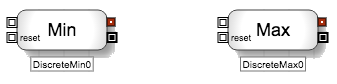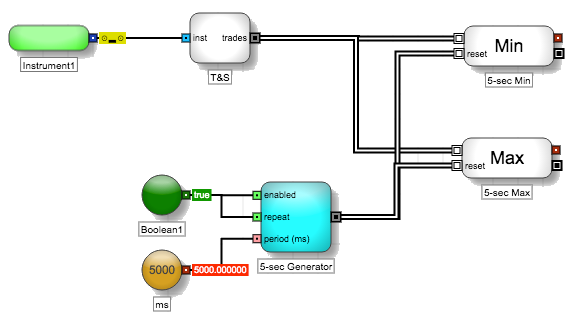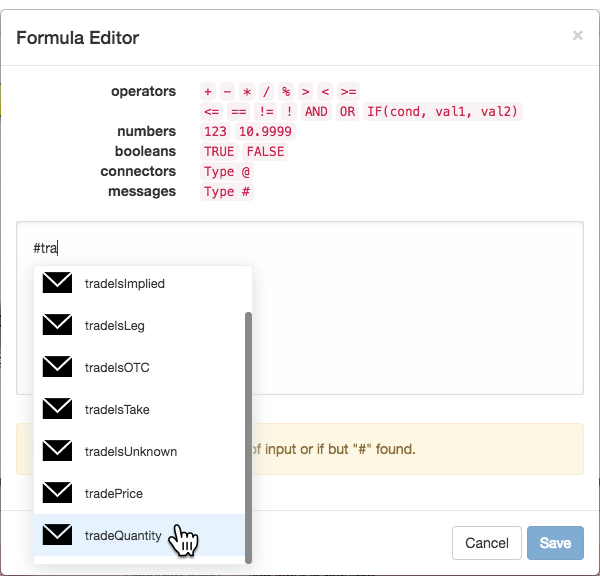Discrete blocks

# Discrete Min/Max blocksThe Discrete Min and Discrete Max blocks output the minimum and maximum values, respectively, of their internal numeric collections. Each time one of the blocks receives a discrete event message, it adds a user-specified numeric value to its internal collection of values and then outputs either the minimum or maximum values in the collection.

The blocks process discrete event messages as follows:

1. A discrete event message triggers the Discrete Min/Max block.
2. When triggered, the Discrete Min/Max block retrieves a numeric value specified by the user using a formula in Block Properties. The user may extract a numeric value contained within the input message or retrieve a value unrelated to the message.
3. The extracted value is added to the block's data collection.
4. The block then determines the Minimum/Maximum value among its collection. The result is sent through the blocks numeric output port.
5. After the evaluation, it passes on the original message unchanged through its discrete event message output port.

For example, suppose a Discrete Max block stores the value of the trade quantity from each incoming discrete event message from a Time and Sales block. As trades occurred, the Discrete Max block stored the values, [5, 10, 5, 25, 50, 10]. If the Discrete Max block receives its next message from the Time and Sales block with a trade quantity of 8, the block would output the value 50 because it represents the maximum value stored by the block. A Discrete Min block with the same values would return 5.

You can also set up the Discrete Min/Max block to delete its internal data collection and reset its output to NaN (Not A Number) when a discrete event message triggers the reset port.

Example Output minimum and maximum trade quantities in a five-second spanThe Discrete Min and Discrete Max blocks would define the following formulas in the Formula Editor.## Block properties

To customize the block, update the Block Properties.

Property Description
Name Name to display beneath the block on the ADL canvas
Formula

Equation used to calculate the value of the block

The edit link opens the Formula Editor.